# Error Detection Hamming Codes 1 Error detecting codes

• Slides: 17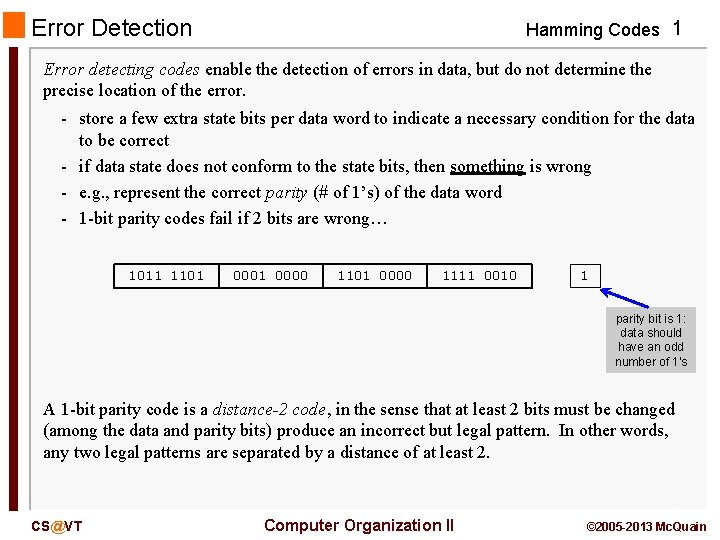Error Detection Hamming Codes 1 Error detecting codes enable the detection of errors in data, but do not determine the precise location of the error. - store a few extra state bits per data word to indicate a necessary condition for the data to be correct - if data state does not conform to the state bits, then something is wrong - e. g. , represent the correct parity (# of 1’s) of the data word - 1 -bit parity codes fail if 2 bits are wrong… 1011 1101 0000 1111 0010 1 parity bit is 1: data should have an odd number of 1's A 1 -bit parity code is a distance-2 code, in the sense that at least 2 bits must be changed (among the data and parity bits) produce an incorrect but legal pattern. In other words, any two legal patterns are separated by a distance of at least 2. [email protected] Computer Organization II © 2005 -2013 Mc. Quain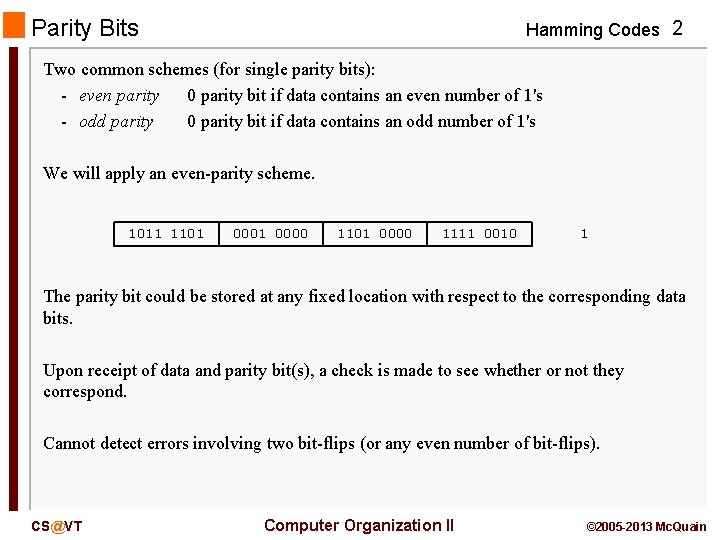Parity Bits Hamming Codes 2 Two common schemes (for single parity bits): - even parity 0 parity bit if data contains an even number of 1's - odd parity 0 parity bit if data contains an odd number of 1's We will apply an even-parity scheme. 1011 1101 0000 1111 0010 1 The parity bit could be stored at any fixed location with respect to the corresponding data bits. Upon receipt of data and parity bit(s), a check is made to see whether or not they correspond. Cannot detect errors involving two bit-flips (or any even number of bit-flips). [email protected] Computer Organization II © 2005 -2013 Mc. QuainHamming (7, 4) Code Hamming Codes 3 Richard Hamming (1950) described a method for generating minimum-length errordetecting codes. Here is the (7, 4) Hamming code for 4 -bit words: Say we receive the data word 0100 and parity bits 011. Those do not match (see the table). Therefore, we know an error has occurred. But where? [email protected] Computer Organization II Data bits d 4 d 3 d 2 d 1 Parity bits P 3 p 2 p 1 0000 0001 011 0010 101 0011 110 0100 110 0101 0110 0111 000 111 100 1010 1011 001 1100 001 1101 010 1110 100 1111 © 2005 -2013 Mc. Quain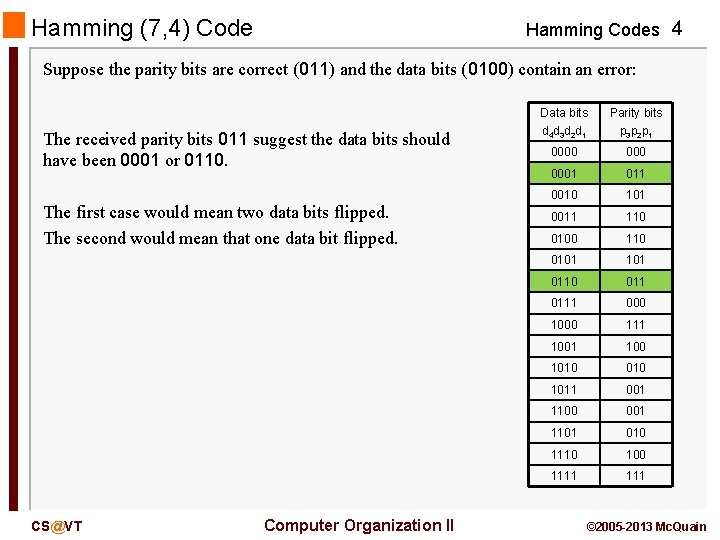Hamming (7, 4) Code Hamming Codes 4 Suppose the parity bits are correct (011) and the data bits (0100) contain an error: The received parity bits 011 suggest the data bits should have been 0001 or 0110. The first case would mean two data bits flipped. The second would mean that one data bit flipped. [email protected] Computer Organization II Data bits d 4 d 3 d 2 d 1 Parity bits p 3 p 2 p 1 0000 0001 011 0010 101 0011 110 0100 110 0101 0110 0111 000 111 100 1010 1011 001 1100 001 1101 010 1110 100 1111 © 2005 -2013 Mc. QuainHamming (7, 4) Code Hamming Codes 5 Suppose the data bits (0100) are correct and the parity bits (011) contain an error: The received data bits 0100 would match parity bits 110. That would mean two parity bits flipped. If we assume that only one bit flipped, we can conclude the correction is that the data bits should have been 0110. If we assume that two bits flipped, we have two equally likely candidates for a correction, and no way to determine which would have been the correct choice. [email protected] Computer Organization II Data bits d 4 d 3 d 2 d 1 Parity bits p 3 p 2 p 1 0000 0001 011 0010 101 0011 110 0100 110 0101 0110 0111 000 111 100 1010 1011 001 1100 001 1101 010 1110 100 1111 © 2005 -2013 Mc. Quain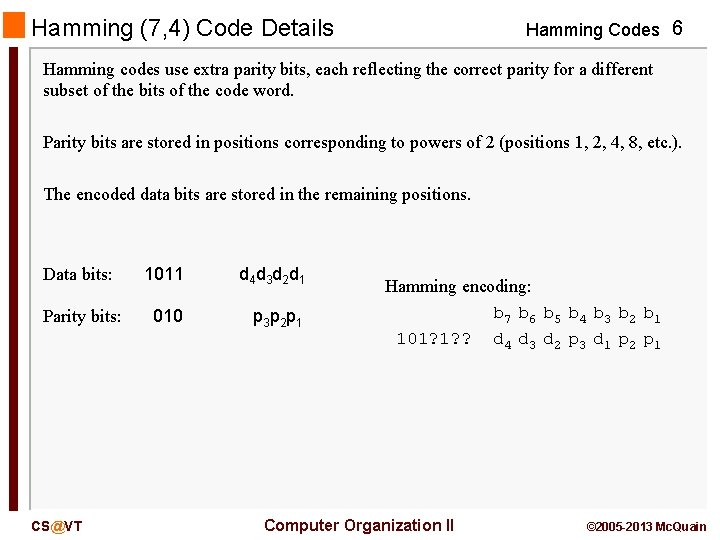Hamming (7, 4) Code Details Hamming Codes 6 Hamming codes use extra parity bits, each reflecting the correct parity for a different subset of the bits of the code word. Parity bits are stored in positions corresponding to powers of 2 (positions 1, 2, 4, 8, etc. ). The encoded data bits are stored in the remaining positions. Data bits: 1011 d 4 d 3 d 2 d 1 Parity bits: 010 p 3 p 2 p 1 [email protected] Hamming encoding: b 7 b 6 b 5 b 4 b 3 b 2 b 1 101? 1? ? d 4 d 3 d 2 p 3 d 1 p 2 p 1 Computer Organization II © 2005 -2013 Mc. QuainHamming Code Details Hamming Codes 7 But, how are the parity bits defined? - p 1: all higher bit positions k where the 20 bit is set (1's bit) - p 2: all higher bit positions k where the 21 bit is set (2's bit) … - pn: all higher bit positions k where the 2 n bit is set Hamming encoding: b 111 b 110 b 101 b 100 b 011 b 010 b 001 101? 1? ? d 4 d 3 d 2 p 3 d 1 p 2 p 1 This means that each data bit is used to define at least two different parity bits; that redundancy turns out to be valuable. [email protected] Computer Organization II © 2005 -2013 Mc. Quain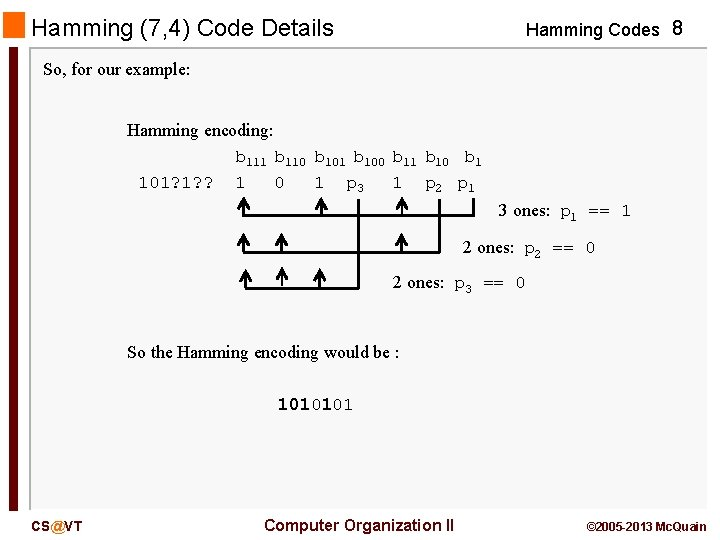Hamming (7, 4) Code Details Hamming Codes 8 So, for our example: Hamming encoding: b 111 b 110 b 101 b 100 b 11 b 10 b 1 101? 1? ? 1 0 1 p 3 1 p 2 p 1 3 ones: p 1 == 1 2 ones: p 2 == 0 2 ones: p 3 == 0 So the Hamming encoding would be : 1010101 [email protected] Computer Organization II © 2005 -2013 Mc. Quain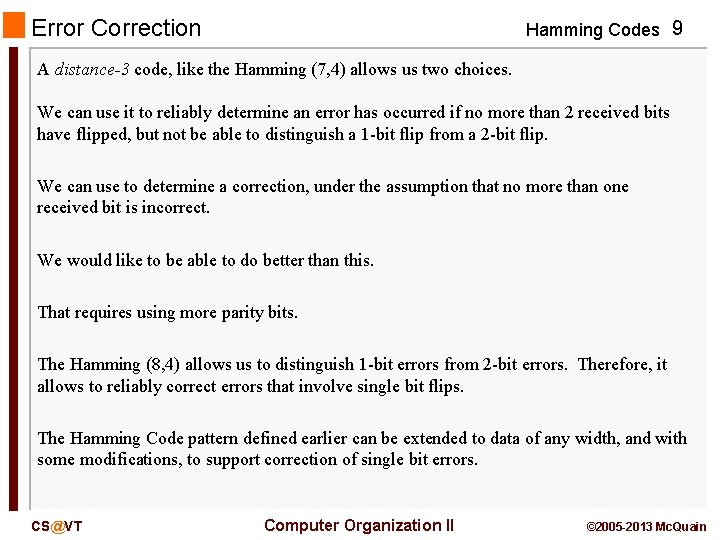Error Correction Hamming Codes 9 A distance-3 code, like the Hamming (7, 4) allows us two choices. We can use it to reliably determine an error has occurred if no more than 2 received bits have flipped, but not be able to distinguish a 1 -bit flip from a 2 -bit flip. We can use to determine a correction, under the assumption that no more than one received bit is incorrect. We would like to be able to do better than this. That requires using more parity bits. The Hamming (8, 4) allows us to distinguish 1 -bit errors from 2 -bit errors. Therefore, it allows to reliably correct errors that involve single bit flips. The Hamming Code pattern defined earlier can be extended to data of any width, and with some modifications, to support correction of single bit errors. [email protected] Computer Organization II © 2005 -2013 Mc. Quain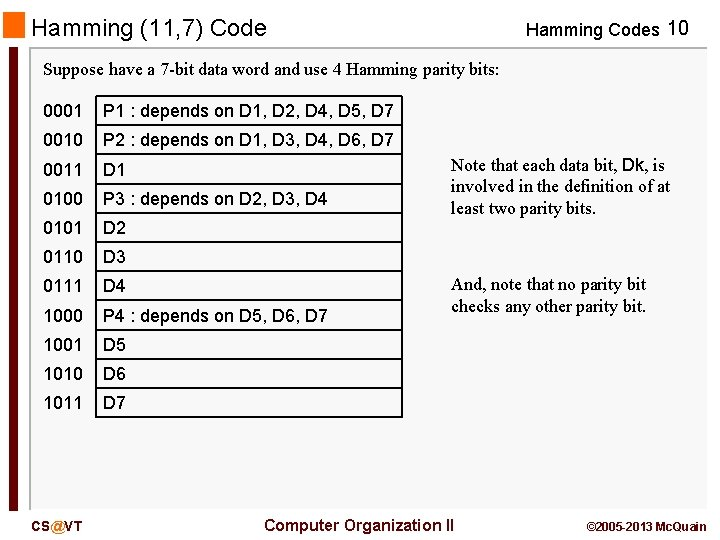Hamming (11, 7) Code Hamming Codes 10 Suppose have a 7 -bit data word and use 4 Hamming parity bits: 0001 P 1 : depends on D 1, D 2, D 4, D 5, D 7 0010 P 2 : depends on D 1, D 3, D 4, D 6, D 7 0011 D 1 0100 P 3 : depends on D 2, D 3, D 4 0101 D 2 0110 D 3 0111 D 4 1000 P 4 : depends on D 5, D 6, D 7 1001 D 5 1010 D 6 1011 D 7 [email protected] Note that each data bit, Dk, is involved in the definition of at least two parity bits. And, note that no parity bit checks any other parity bit. Computer Organization II © 2005 -2013 Mc. Quain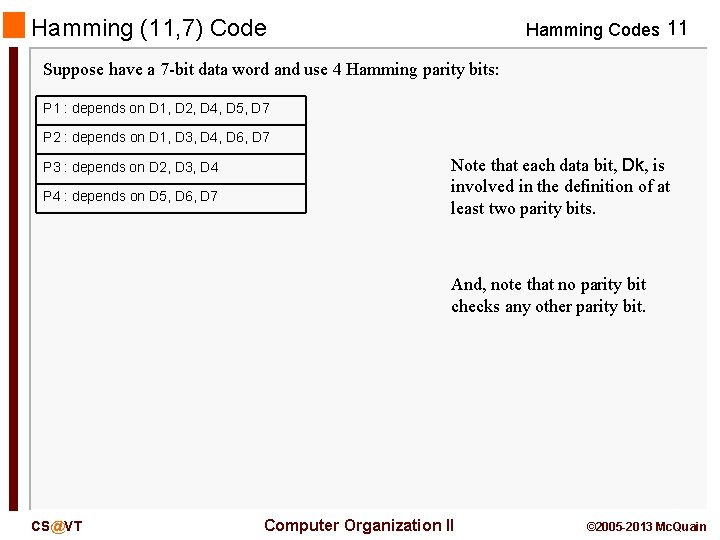Hamming (11, 7) Code Hamming Codes 11 Suppose have a 7 -bit data word and use 4 Hamming parity bits: P 1 : depends on D 1, D 2, D 4, D 5, D 7 P 2 : depends on D 1, D 3, D 4, D 6, D 7 P 3 : depends on D 2, D 3, D 4 P 4 : depends on D 5, D 6, D 7 Note that each data bit, Dk, is involved in the definition of at least two parity bits. And, note that no parity bit checks any other parity bit. [email protected] Computer Organization II © 2005 -2013 Mc. Quain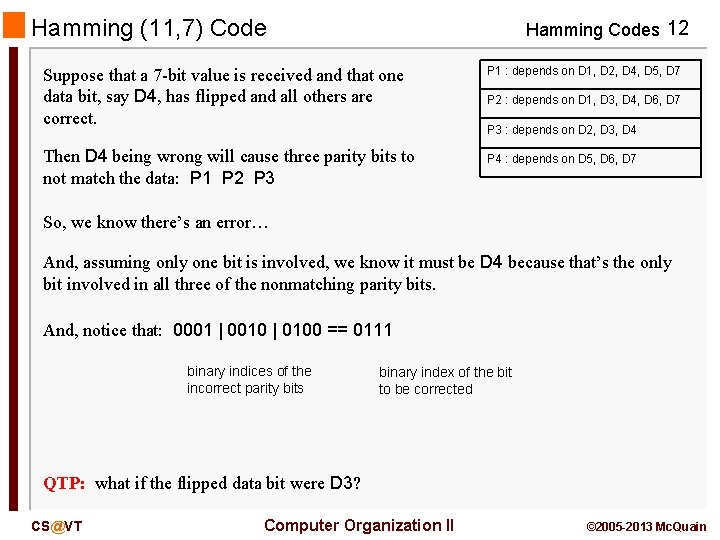Hamming (11, 7) Code Hamming Codes 12 Suppose that a 7 -bit value is received and that one data bit, say D 4, has flipped and all others are correct. Then D 4 being wrong will cause three parity bits to not match the data: P 1 P 2 P 3 P 1 : depends on D 1, D 2, D 4, D 5, D 7 P 2 : depends on D 1, D 3, D 4, D 6, D 7 P 3 : depends on D 2, D 3, D 4 P 4 : depends on D 5, D 6, D 7 So, we know there’s an error… And, assuming only one bit is involved, we know it must be D 4 because that’s the only bit involved in all three of the nonmatching parity bits. And, notice that: 0001 | 0010 | 0100 == 0111 binary indices of the incorrect parity bits binary index of the bit to be corrected QTP: what if the flipped data bit were D 3? [email protected] Computer Organization II © 2005 -2013 Mc. Quain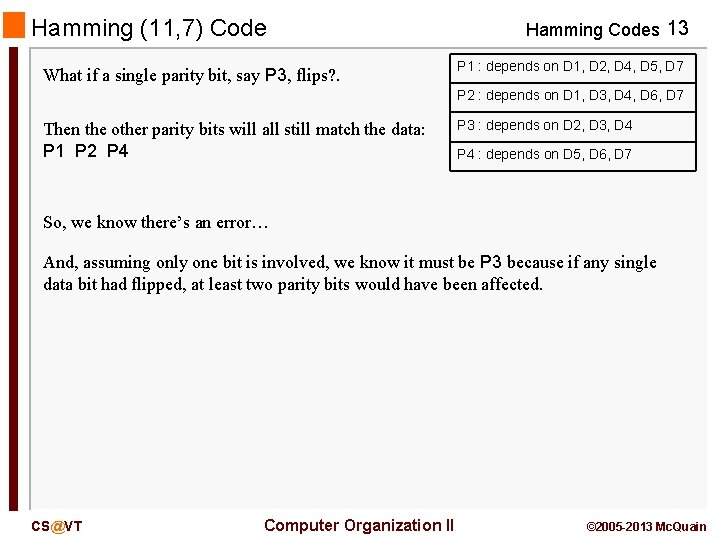Hamming (11, 7) Code What if a single parity bit, say P 3, flips? . Hamming Codes 13 P 1 : depends on D 1, D 2, D 4, D 5, D 7 P 2 : depends on D 1, D 3, D 4, D 6, D 7 Then the other parity bits will all still match the data: P 1 P 2 P 4 P 3 : depends on D 2, D 3, D 4 P 4 : depends on D 5, D 6, D 7 So, we know there’s an error… And, assuming only one bit is involved, we know it must be P 3 because if any single data bit had flipped, at least two parity bits would have been affected. [email protected] Computer Organization II © 2005 -2013 Mc. Quain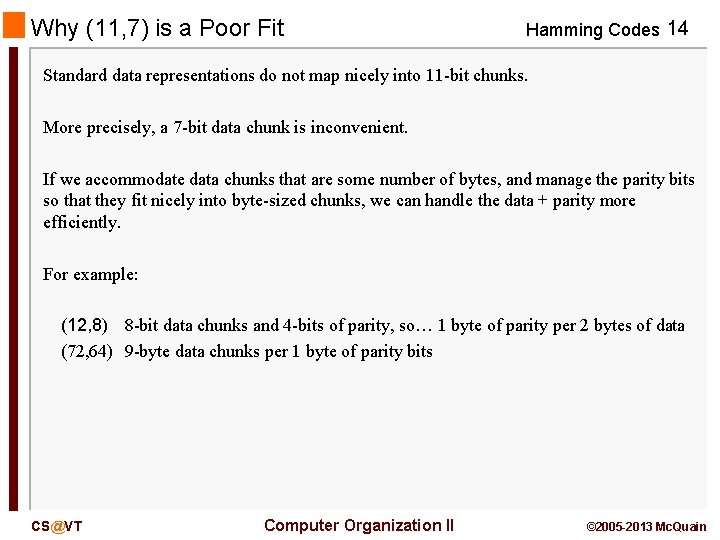Why (11, 7) is a Poor Fit Hamming Codes 14 Standard data representations do not map nicely into 11 -bit chunks. More precisely, a 7 -bit data chunk is inconvenient. If we accommodate data chunks that are some number of bytes, and manage the parity bits so that they fit nicely into byte-sized chunks, we can handle the data + parity more efficiently. For example: (12, 8) 8 -bit data chunks and 4 -bits of parity, so… 1 byte of parity per 2 bytes of data (72, 64) 9 -byte data chunks per 1 byte of parity bits [email protected] Computer Organization II © 2005 -2013 Mc. Quain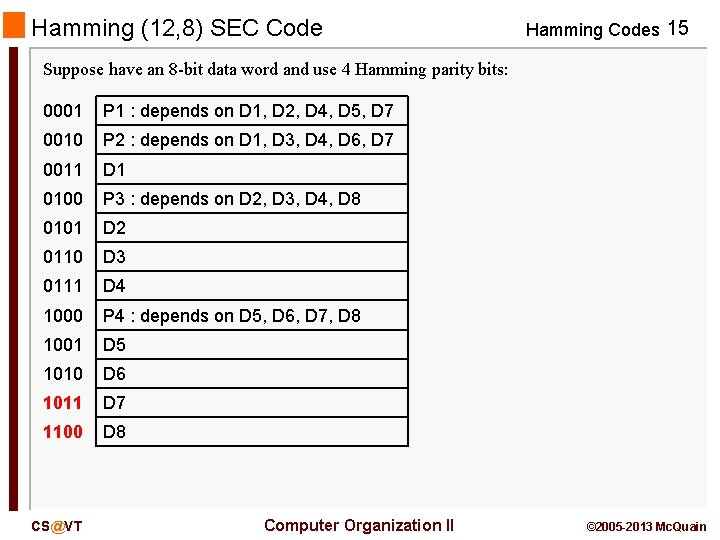Hamming (12, 8) SEC Code Hamming Codes 15 Suppose have an 8 -bit data word and use 4 Hamming parity bits: 0001 P 1 : depends on D 1, D 2, D 4, D 5, D 7 0010 P 2 : depends on D 1, D 3, D 4, D 6, D 7 0011 D 1 0100 P 3 : depends on D 2, D 3, D 4, D 8 0101 D 2 0110 D 3 0111 D 4 1000 P 4 : depends on D 5, D 6, D 7, D 8 1001 D 5 1010 D 6 1011 D 7 1100 D 8 [email protected] Computer Organization II © 2005 -2013 Mc. QuainHamming (12, 8) SEC Code Correction Hamming Codes 16 Suppose we receive the bits: 0 1 1 1 0 How can we determine whether it's correct? Check the parity bits and see which, if any are incorrect. If they are all correct, we must assume the string is correct. Of course, it might contain so many errors that we can't even detect their occurrence, but in that case we have a communication channel that's so noisy that we cannot use it reliably. 0 1 1 1 0 OK OK WRONG So, what does that tell us, aside from that something is incorrect? Well, if we assume there's no more than one incorrect bit, we can say that because the incorrect parity bits are in positions 4 (0100) and 8 (1000), the incorrect bit must be in position 12 (1100). [email protected] Computer Organization II © 2005 -2013 Mc. Quain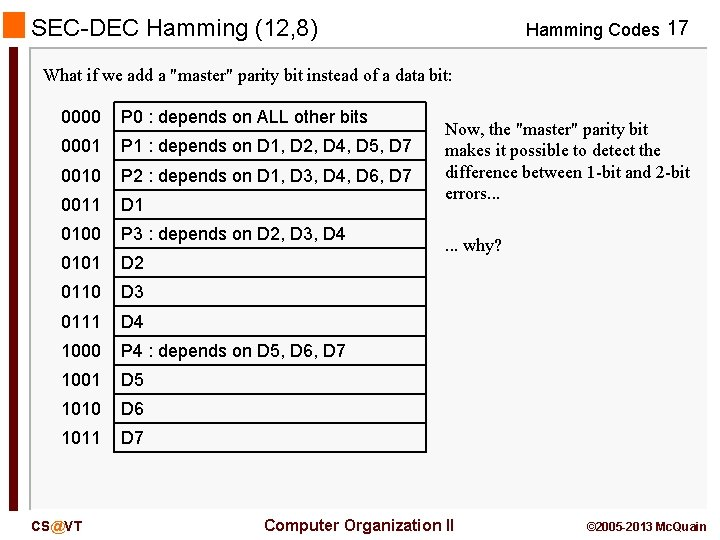SEC-DEC Hamming (12, 8) Hamming Codes 17 What if we add a "master" parity bit instead of a data bit: 0000 P 0 : depends on ALL other bits 0001 P 1 : depends on D 1, D 2, D 4, D 5, D 7 0010 P 2 : depends on D 1, D 3, D 4, D 6, D 7 0011 D 1 0100 P 3 : depends on D 2, D 3, D 4 0101 D 2 0110 D 3 0111 D 4 1000 P 4 : depends on D 5, D 6, D 7 1001 D 5 1010 D 6 1011 D 7 [email protected] Now, the "master" parity bit makes it possible to detect the difference between 1 -bit and 2 -bit errors. . . why? Computer Organization II © 2005 -2013 Mc. Quain